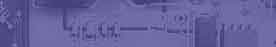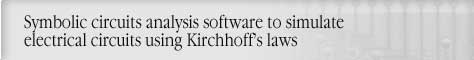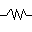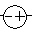AC Electrical Devices & schematics symblosCircuit analysis Tutorial
AKNM Circuit Magic- circuit analysis software

AC Electrical Devices & schematics symblos

Electronic component are classed into either being Passive devices or Active devices.

By using schematics symbols we can represent real-life devices.Resistance -This is a resistance, measured in units ohms, . Most often it will be a resistor.Inductor - A schematics symbol inserted into an electrical circuit to introduce a specified inductance.. Most often it will be a coil.

Inductance-
The property of a circuit which impedes a change in current. Inductors are the usual source of inductance. Inductance is measured in henrys. In electronic circuits, the usual measure of inductance is henrys (H), milihenrys (mH) or microhenrys (µH), 1, 1e-3 or 1e-6 henrys respectively.orCapacitor - A schematics symbol inserted into an electrical circuit to introduce a specified capacitance.

Definition: The property of a circuit which impedes a change in voltage. Capacitors are the usual source of capacitance. Capacitance is measured in farads in honor of Michael Faraday. In electronic circuits, the usual measure of capacitance is microfarads (µF) or picofarads (pF), 1e-6 or 1e-12 farads respectively.This is a AC voltage source, with a voltage of , measured in units of volts, V. The most common AC Voltage Source you will see will be a home unit. .A device inserted into an electrical circuit to introduce a specified alternating current (AC). An AC current has a magnitude, phase and frequency.